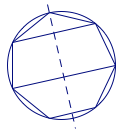# [竞赛] 组合几何中的论证与构造

（IMO-56）设$S$为平面上一个有限点集，如果对$S$中任意两个不同的点$A$、$B$，都存在$S$中的一点$C$，使得$AC=BC$，我们称$S$为平衡的．如果对$S$中的任意三个不同点$A$、$B$、$C$，都不存在$S$中的一点$P$，满足$PA=PB=PC$，我们称$S$是无中心的．

（1）证明：对每个正整数$n\geqslant 3$，均存在一个由$n$个点构成的平衡的点集；

（2）确定所有的正整数$n\geqslant 3$，使得存在一个由$n$个点构成的平衡且无中心的点集．

（1）证明    从简单情形着手考虑．

（2）    只有当$n$为不小于$3$的奇数时，存在由$n$个点构成的平衡且无中心的点集，证明如下．